Design Guide Details
Design Guide Details
Date: 16-02-17

# VLSI Design: Noise analysis in Amplifier Circuits

Noise analysis is very important in many analog and RF receiver circuit design since the received signals are very weak. In case of differential amplifiers the noise appear as common mode signal and hence is rejected. However is single stage amplifiers the contribution of various circuit elements to noise must be taken into consideration. Resistors, MOSFETs and Diodes are the common sources of noise in analog circuits. In order to perform noise analysis we need to have accurate noise models for various elements used in the design.
Since noise voltages and currents are small in magnitude, their influence on the circuit can be obtained through small signal analysis of the circuit. As a result, the noise voltages and currents can be added to the small signal model of the circuit.

There are several different kinds of noise sources that can be found in semiconductor devices. Perhaps the most important is the Thermal Noise.

Rms noise due to sum of two uncorrelated noise sources e1 and e2 will be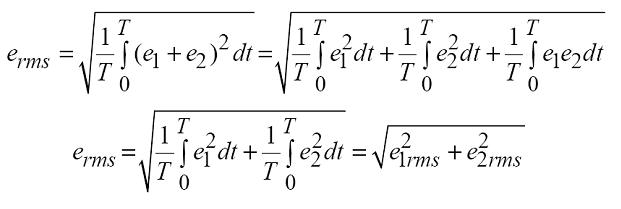Thermal noise results from random motion of charge carriers within a conductor or a semiconductor due to the presence of scattering centers.

Any resistor can be modeled as a noiseless resistor in series with a rms noise voltage source as shown below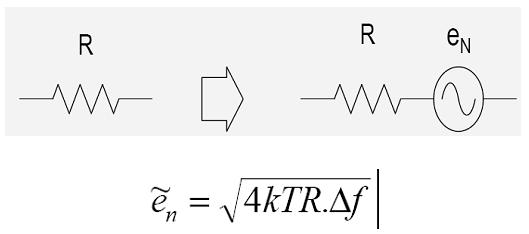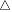f is the bandwidth over which noise is measured. The thermal noise has a uniform spectral density and is termed as white noise.
The noise in a MOSFET arises from thermal noise due to channel resistance and flicker noise component due carrier trapping at the oxide interface.

Thermal noise current in MOSFET is given by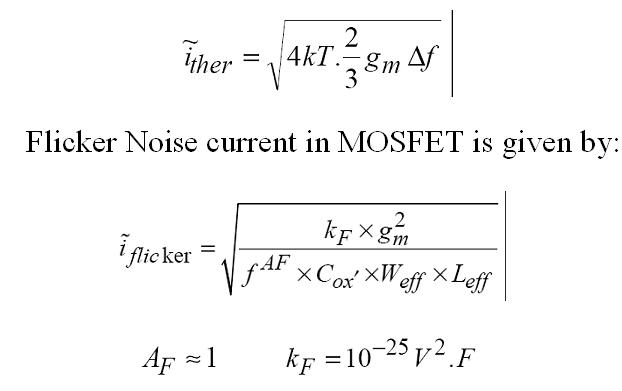Since noise voltages and currents are small, they can be represented as part of small signal model as shown below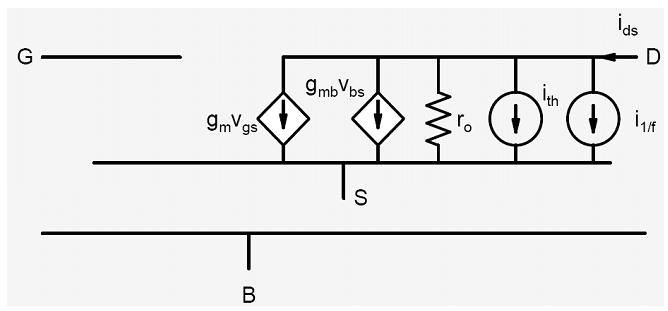Noise Analysis of Common Source Amplifier with resistive load: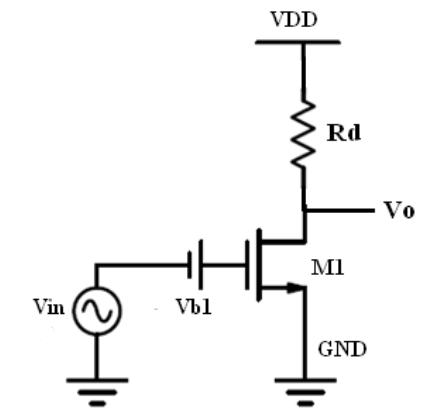The small signal equivalent of above circuit including noise sources is given by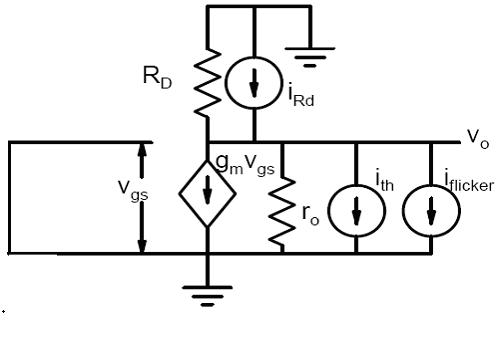To obtain the total Noise Voltage at the output we can consider one source at a time and determine the output. The RMS value of total noise voltage is given below.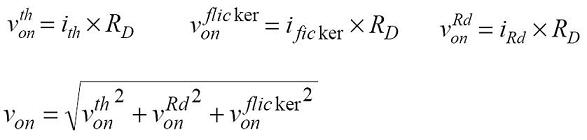Noise Analysis of CS Amplifier with active Load:

Generally CS amplifier with active load is used to increase the small signal Gain. To obtain large gain with resistive load we need large resistor which consume lot of area and also introduce noise. Schematic of CS Amplifier with Active load is shown below. The PMOS load appear as resistor with value rop at the output for small signal analysis.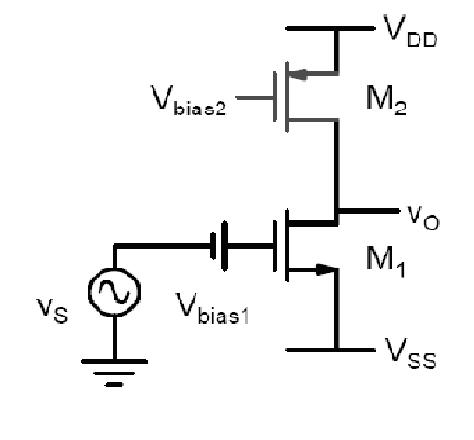The small signal equivalent of above circuit with noise sources is given by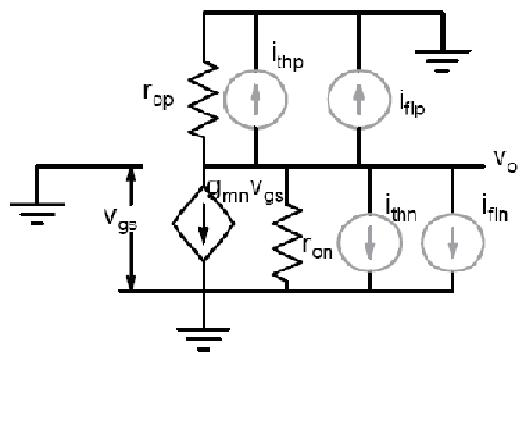Where ith represent thermal noise current and ifl represent flicker noise current. The noise current can be calculated as follows.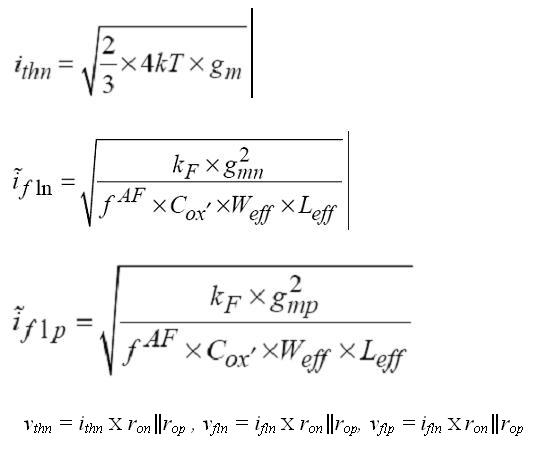The RMS value of output noise voltage can be calculated as explained in previous section with resistor load. Most of the time it is the input referred noise which is of greater interest. This can be obtained just by dividing the above obtained noise voltage by the Gain of the amplifier stage.